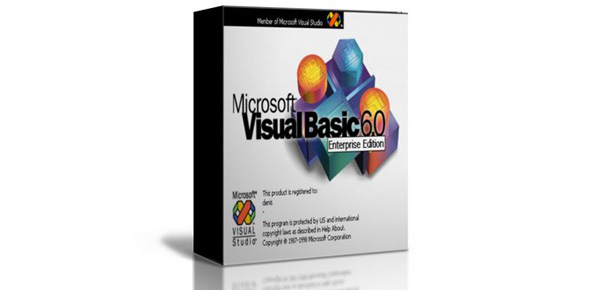# Random Numbers With Rnd

16 Questions | Attempts: 126
ShareSettingsStudy the RandomNumber. Frm code and its output first. Use your answers that you put on paper from the assignment. After you are finished, read the corrections and the explanations that go with them. Even if you got all the answers correct, please read the explanations that are provided below the problem. If you did not get 100%, click on Try Again at the bottom. Correct your paper answers as well.

• 1.
When you run your RandomNumber.frm program, do you get the same exact results as the example shown on the direction sheet?
• A.

Yes

• B.

No

• 2.
Why or why not?
• A.

The output is the same because I did it correctly.

• B.

The output is different because the numbers are random.

• 3.
What line in the code makes cmdLessOne produce a decimal?
• A.

Dim LessOne As Single

• B.

Randomize

• C.

LessOne = Rnd

• D.

LblLessOne = LessOne

• 4.
What line in the code makes cmdTen produce a decimal?
• A.

Dim LessTen As Single

• B.

Randomize

• C.

LessTen = 10 * Rnd

• D.

LblLessTen = LessTen

• 5.
What line in the code makes cmdFive produce an integer?
• A.

Dim UpTo5 As Integer

• B.

Randomize

• C.

UpTo5 = 5 * Rnd

• D.

LblUpTo5 = UpTo5

• 6.
What line in the code makes cmd100 produce an integer?
• A.

Dim UpTo100 As Integer

• B.

Randomize

• C.

UpTo100 = 100 * Rnd

• D.

LblUpTo100 = UpTo100

• 7.
What line of code guarantees that the results will be truly random?
• A.

Dim UpTo100 As Integer

• B.

Randomize

• C.

UpTo100 = 100 * Rnd

• D.

LblUpTo100 = UpTo100

• 8.
What is the keyword in Visual Basic that produces a random number between 0 and 1?
• A.

Randomize

• B.

Rnd

• C.

LessOne

• D.

Random

• 9.
What type of numbers exist between 0 and 1?
• A.

Integers

• B.

Decimals (Single)

• 10.
When you multiply a number between 0 and 1 by 10, how many digits are to the left of the decimal point?
• A.

0

• B.

1

• C.

2

• D.

10

• 11.
Describe the number that will be stored in memory because of this line of code in cmdTen. LessTen = 10 * Rnd
• A.

A random decimal number less than 10 such as 2.3482 or 9.3627

• B.

A random integer less than 10 such as 2 or 9

• C.

A random decimal number between 0 and 1 such as .23 or .93

• D.

A random integer as small or big as the computer will allow

• 12.
Describe the number that will be stored in the variable, UpTo5, because of this line of code in cmdFive. UpTo5 = 5 * Rnd
• A.

A random decimal less than 5

• B.

A random decimal from 0 to 5

• C.

A random integer from 0 to 5

• D.

A random integer from 0 to 5000

• 13.
What must Rnd be multiplied by to produce a number between 0 and 33?
• A.

300

• B.

.3

• C.

3.3

• D.

33

• E.

13

• 14.
Write the line of code that will put a random number between 0 and 33 in the variable, UpTo33.
• A.

UpTo33 = Rnd

• B.

UpTo33 = 33 * Rnd

• C.

UpTo33 = 10 * Rnd

• D.

UpTo33 = Rnd + 13

• 15.
To have a program user pick the highest number, use a textbox to get the number and multiply Rnd by the numerical variable where that number is stored.
• A.

True

• B.

False

• 16.
Which of these could be the assignment statement for putting the random integer from 0 to the top number the user provided, into a variable in memory, if these declarations are given?Dim Top As IntegerDim UpToTop As Integer
• A.

UpToTop = 10 * Rnd

• B.

UpToTop = Top * Rnd

• C.

UpToX = Top * Rnd

• D.

UpToHigh = Top * Rnd

• E.

UpToX = X * Rnd

## Related TopicsBack to top
×

Wait!
Here's an interesting quiz for you.